# Purpose

In our "Part 1 of Cost Loading" article, we went over creating a new resource for cost loading purposes, assigning it to the activity, and adding costs to those activities. If you haven't seen that article, check it out here as we'll be referencing the schedule file that we created in that article. In this article, we'll go over how to update a cost loaded schedule.

# Overview

When updating a cost loaded schedule, we can break it down into the following three steps:

Step 1: Update the activities

Step 2: Ensure the costs are shown accurately

Step 3: Prepare for the next month's update (store financial period data).

The first two steps are relatively simple. The last step is simple but it can be confusing if you don't know what financial periods are in P6. We'll look at what they are, and how to use them to give you another tool in the tool belt.

Let's get started.

# Instructions

## Step 1 - Updating the Activities

For reference, we'll be working from the file that we cost loaded in the previous article. If you want to download the P6 file, it's attached to the bottom of this article.

To begin, let's look at where we left off in the last article. We have the following schedule where each construction activity is assigned a value of \$5,000.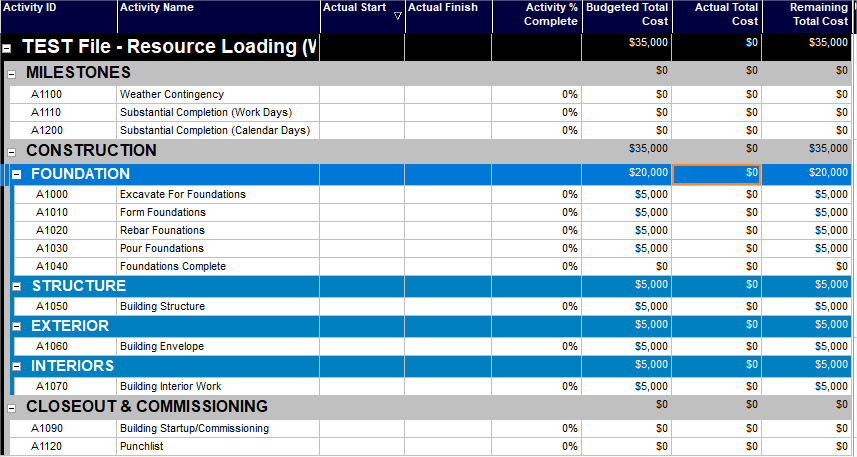Let's progress the first few construction activities with the following status:

• Excavate For Foundations: 100%
• Form Foundations: 100%
• Rebar Foundations: 100%
• Pour Foundations: 50%

You'll notice that when you update the activities, the actual cost will automatically change to reflect the activity % complete. This is because when we set our resource up in the previous article, we used the "Calculate Cost from Units" option, set our cost per unit to \$1, and used the "Budgeted Units" as the place to input our costs. If that's confusing to you, please reference the last article to better understand.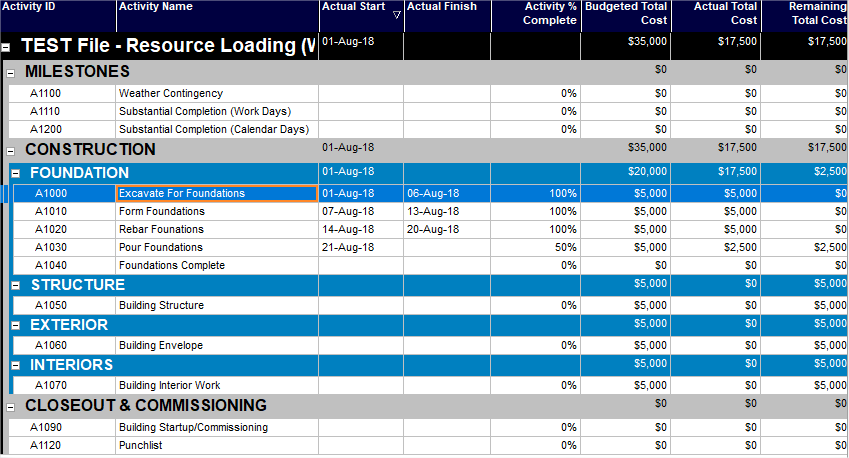As far as updating the activities, it's really this simple. Make sure the activity % complete represents the percentage of billing that you want to apply. Next, we'll verify that our costs our correct.

## Step 2 - Verify Costs are Accurate

The verification process is going to vary by each project. Typically, I'll do a few checks:

1.) Make sure that the budgeted cost column is equal to the at completion total cost column. If you're importing progress or costs from excel, sometimes the remaining costs will get skewed. This error will be made clear if the "At Completion Total Cost" column is different than the "Budgeted Total Cost" column. So just as a quick check, make sure these two columns are equal to one another.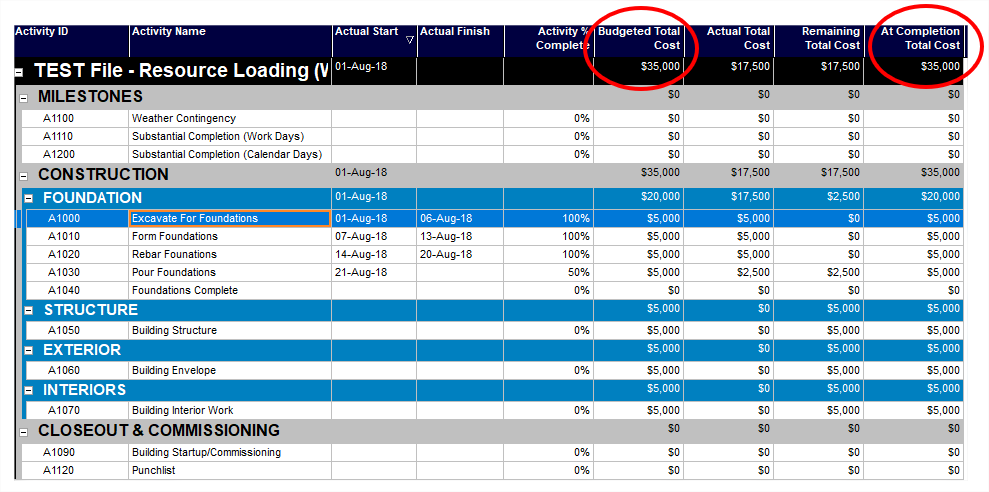2.) Depending on how the specifications define the pay app process, make sure that the values in the schedule align with the pay application (if required).

3.) Export the file and then re-import it into your database to make sure the values don't change change. The worst is when you send the file to the Owner, and they tell you that the values aren't the same as what your database was showing. Often times, the reason is because the "Calculate Costs from Units" option is checked, and the costs are different between the units and the costs columns on the resource assignment.

Let's look at an example of how this could happen. The snapshot below shows the activity that we're going to focus on. As you can see, the budgeted cost is \$5,000 and the actual total cost is \$5,000.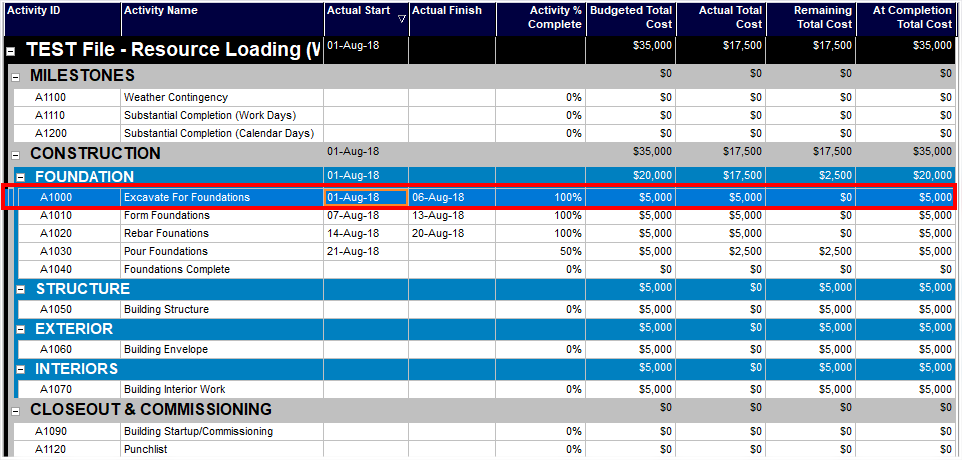However, if we look at the activities details, we can see that the "Budgeted Units" equal 0 and the "Actual Units" equal 0. But our "Calculate Costs from Units" is checked. So what do you think is going to happen when we export the file and import it to a new database?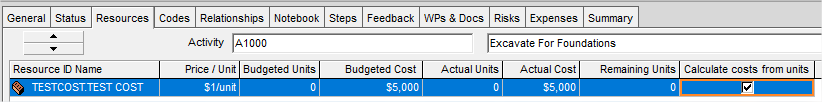After we import the file, you'll notice that our costs were erased on that activity.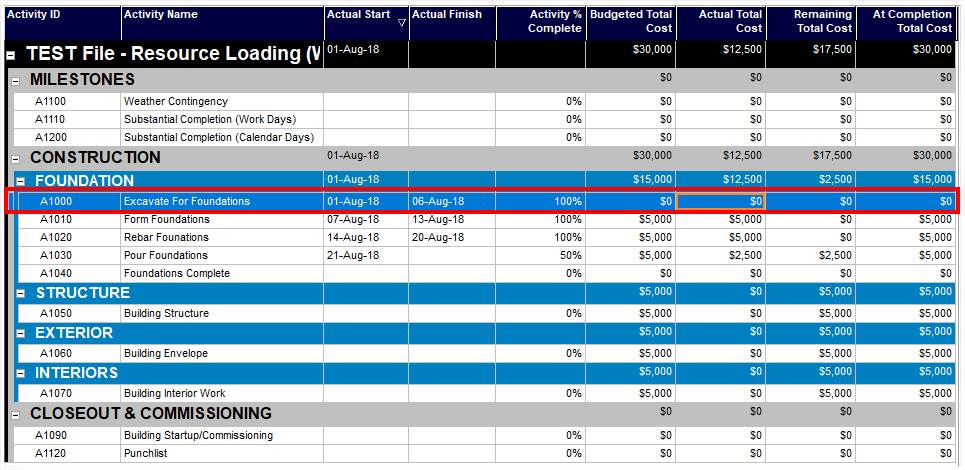In order to fix that, we need to make sure that the Units on the resource assignment is correct, as that's what will be used to make the cost calculation upon the import process.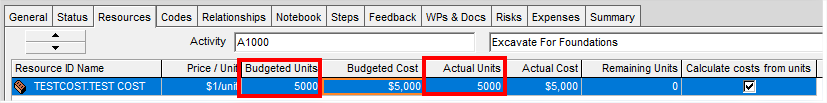## Step 3 - Setup for Next Month

Once our update is complete, we'll need to set the schedule up for next month. I want to show you a column we haven't used yet which is the "Actual Cost This Period" column. You'll notice from the snapshot below that our "Actual Cost This Period" column is equal to the "Actual Total Cost" column.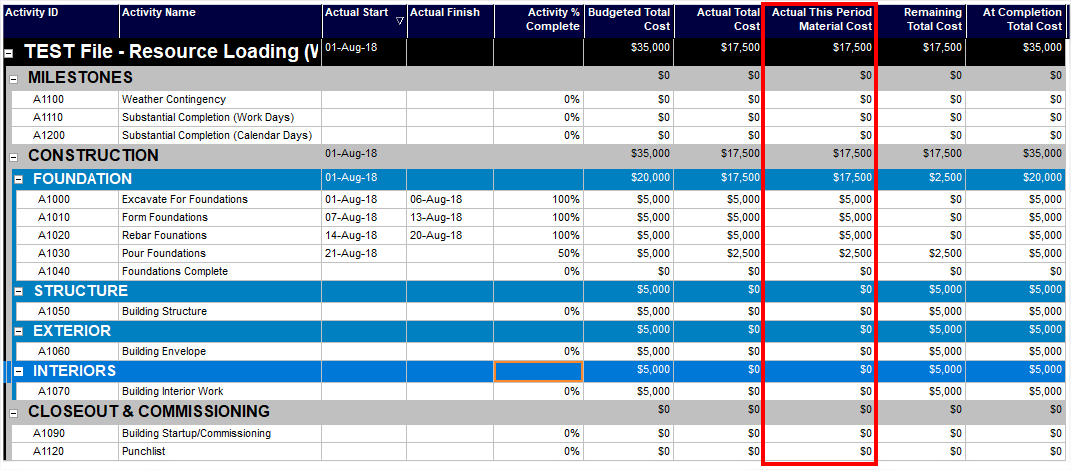When we get to next month, we want that column to reset to zero and only measure the costs that have accrued for the new period. That's where "Stored Financial Periods" come in. This function in P6 will move the values in the "Actual This Period Cost" column to a stored financial period that we'll create. Then, the "Actual This Period Cost" column will reset to \$0 and allow us to accrue new costs for the new period.

So how do we access the stored financial periods? Well first, we have to create them. In order to create them, you have to have admin privileges as they are located under the Admin > Financial Period tab as shown below: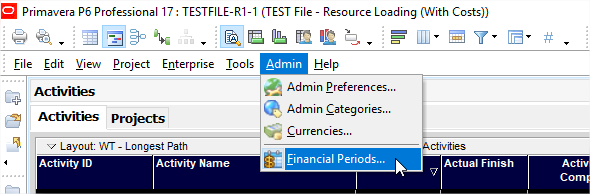When you open up the financial periods, the following window will pop up showing the different financial periods that exist in the schedule.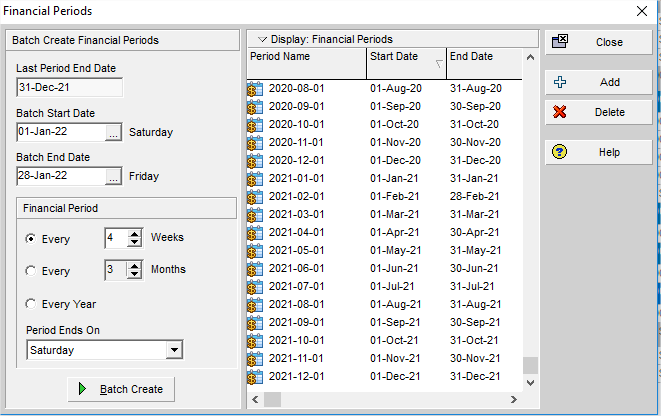If none exist, don't worry, we'll add some right now. Just to back up and understand the financial periods a little better, let's run through an example together. Let's say it is January 2018, it's our very first schedule update, and we have accrued \$35,000 of actual total cost. Our schedule along with our financial periods would look something like this: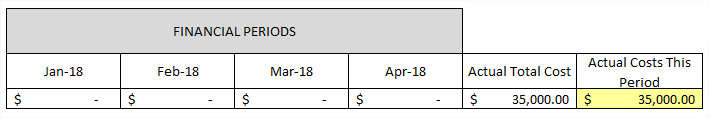You can see that we have no costs in our financial periods as this is our first update. Our "Actual Total Cost" is also equal to our "Actual Costs This Period" at \$35,000. So, we get to the end of January 2018 and it's time to prepare the file for February 2018. We want our "Actual Costs This Period" to reset to \$0. We run the function in P6 to store our period performance to the January 2018 financial period. Let's see what happens: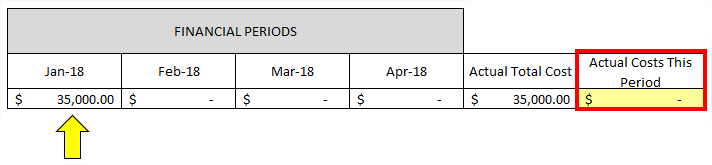You can see that our "Actual Costs This Period" were zeroed out and stored to the January 2018 financial period. This allows for February 2018 to be accrued based on the progress that gets made during that specific month. Let's run through the month of February and see what happens. Let's say we make an additional \$100,000 of progress in the month of February. We get to the end of the month and our schedule looks something like this: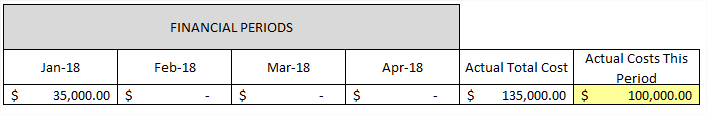You can see that our "Actual Costs This Period" are unique to the February period and have accrued \$100,000. It's now the end of February and it's time to prep the file for March. So we want to store that \$100,000 to the February 2018 financial period. We run the "store financial period" function in P6, and here's what that looks like: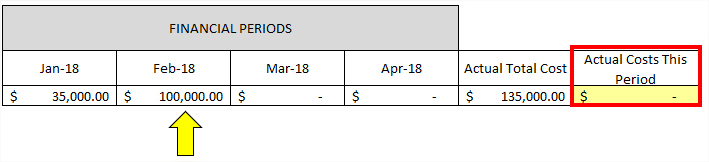The Actual Costs This Period get stored to February 2018. Notice how the "Actual Total Cost" equals the sum of all of the financial periods.

Let's jump back to our exercise and create a batch of financial periods. We have to create the financial periods because those act as the table where the financial information will get stored. In our example, January through April was our "Financial Batch". P6 makes you create the financial period batches where you can store your historical costs.

So when we create a new batch, we need to provide the Batch Start Date, which should coincide with the data dates that you use for each update. For instance, if every month you use the 1st as the data date, your batch start date should be the 1st of the month beginning with the month when the project started. We also need the batch end date which should be the last day of the month or financial period. Financial periods for construction projects typically run every 1 month, which I've shown below. But you can change that if your project is ran differently. Once we provide the information below, we can click "Batch Create".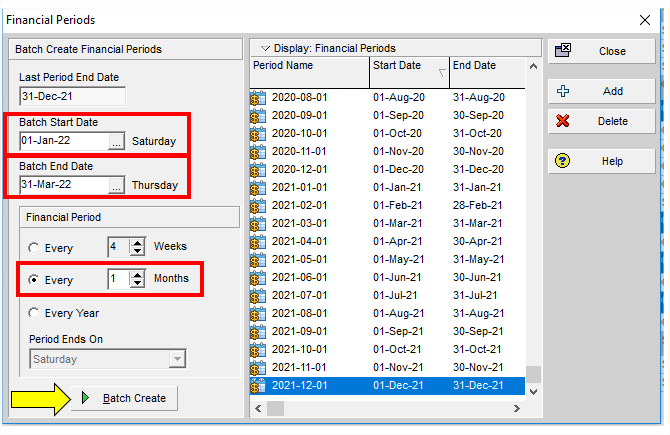You'll see below that three new financial periods were added to the schedule: 2022-01-01, 2022-02-01, and 2022-03-01.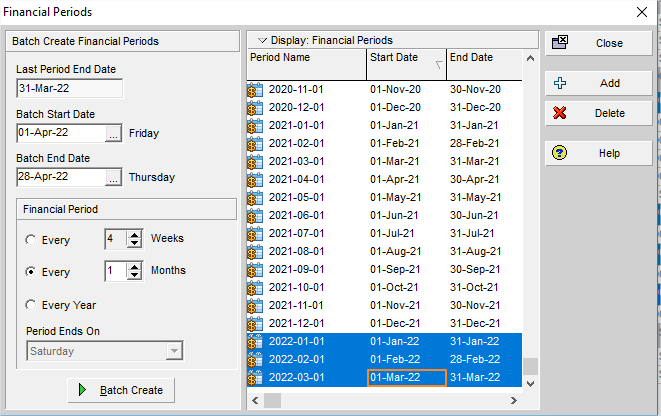Let's close out of this window so we can store our financial period data to the "2022-01-01" period. I want to add a column so that I can show you that we stored the financial period data successfully. In order to access the financial period columns, we have to first go to "Edit" > "User Preferences"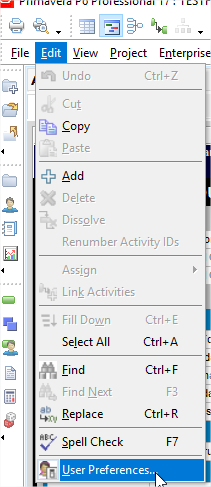Under the "Application" tab, locate the "Columns" section as shown below. Click the "…" to select the first financial period we want to include in our columns.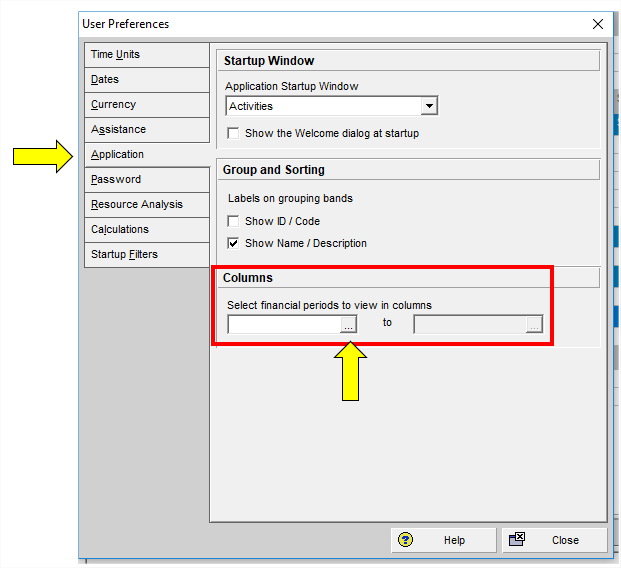I'm going to select the first financial period that we created which was "2022-01-01" as shown below.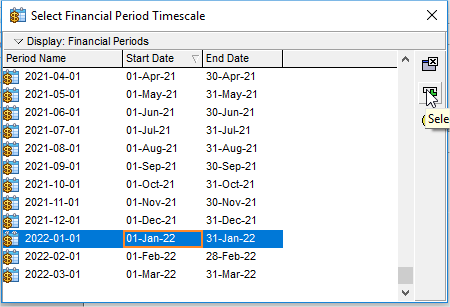We then need to select the last financial period that we want to include in our columns. In my case, I chose the "2022-03-01" period as shown below.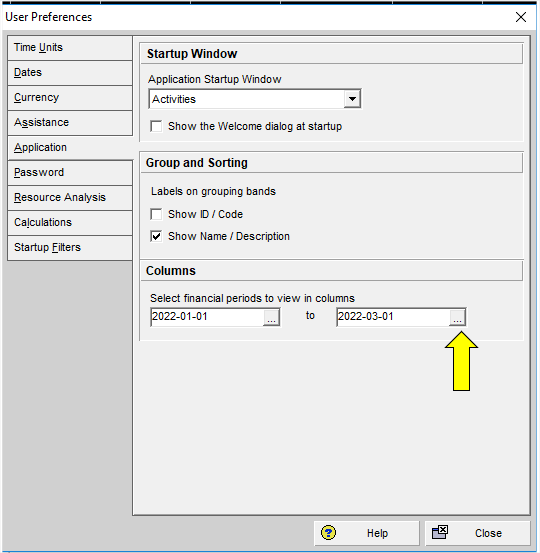Let's close out of this window and now we're going to add a new column to our activities table in P6. You'll notice that there is now a "Financial Period Value" selection under our columns. There's a bunch of different types of data that can get stored, but we want to focus on "Actual Material Cost" for 2022-01-01 as the resource we are using is a material resource.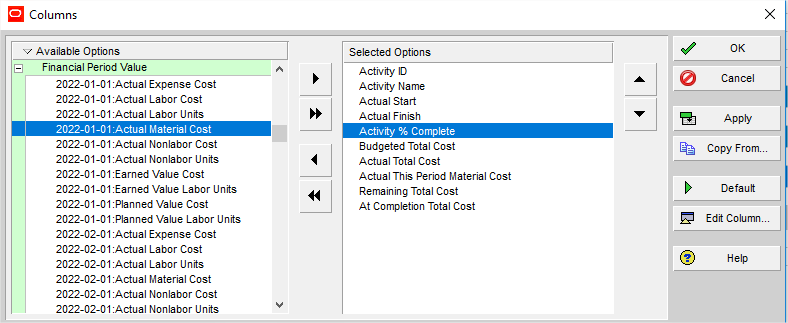So now, our activities table should look like this: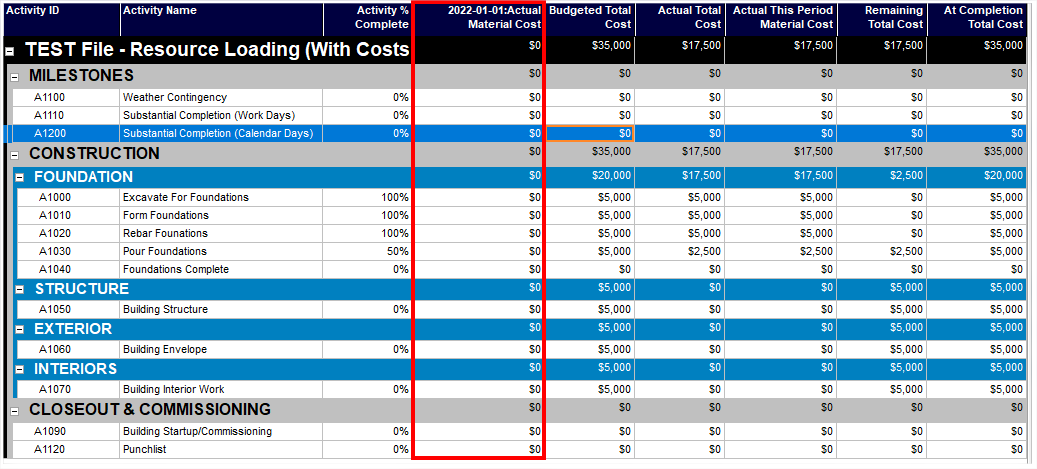We have our new financial period column with \$0. We now want to store our "Actual This Period Material Cost" of \$17,500 to the "2022-01-01:Actual Material Cost" financial period. We do this by going to "Tools" > "Store Period Performance" as shown below: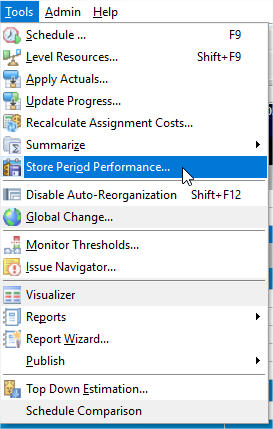Choose the financial period where you want to store the information and then click "Store Now".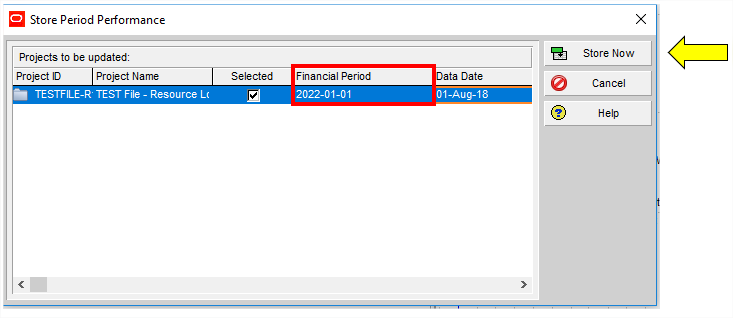You can see now that our "Actual This Period Material Cost" was zeroed out and stored to the financial period that we selected.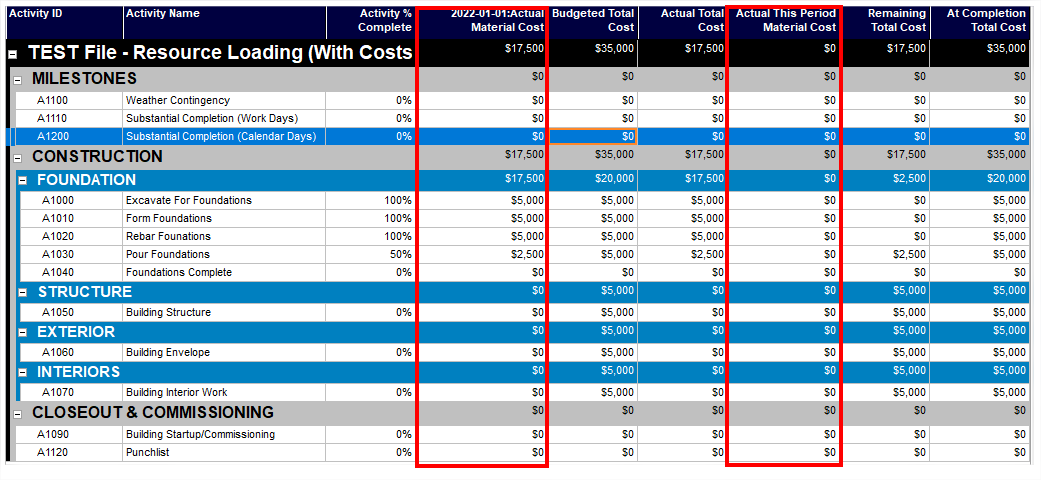We are now ready to start the next month's update where the "Actual This Period Material Cost" will accrue uniquely to the new month!

# Conclusion

I'm aware that if you're unfamiliar with this process, it can be really overwhelming. I would encourage you to go through the exercise yourself several times and familiarize yourself with the process as it becomes easier with repetition. Feel free to leave a comment or question below! I'd love to hear from you.

Happy Scheduling!